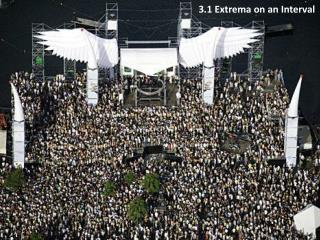# 3.1 Extrema on an Interval - PowerPoint PPT PresentationDownload Presentation3.1 Extrema on an Interval

Presentation Description
Download Presentation## 3.1 Extrema on an Interval

- - - - - - - - - - - - - - - - - - - - - - - - - - - E N D - - - - - - - - - - - - - - - - - - - - - - - - - - -
##### Presentation Transcript

1. 3.1 Extrema on an Interval

2. The mileage of a certain car can be approximated by: At what speed should you drive the car to obtain the best gas mileage? The textbook gives the following example at the start of chapter 4: Of course, this problem isn’t entirely realistic, since it is unlikely that you would have an equation like this for your car.

3. We could solve the problem graphically:

4. We could solve the problem graphically: On the TI-89, we use F5 (math), 4: Maximum, choose lower and upper bounds, and the calculator finds our answer.

5. The car will get approximately 32 miles per gallon when driven at 38.6 miles per hour. We could solve the problem graphically: On the TI-89, we use F5 (math), 4: Maximum, choose lower and upper bounds, and the calculator finds our answer.

6. Notice that at the top of the curve, the horizontal tangent has a slope of zero. Traditionally, this fact has been used both as an aid to graphing by hand and as a method to find maximum (and minimum) values of functions.

7. Even though the graphing calculator and the computer have eliminated the need to routinely use calculus to graph by hand and to find maximum and minimum values of functions, we still study the methods to increase our understanding of functions and the mathematics involved. Absolute extreme values are either maximum or minimum points on a curve. They are sometimes called global extremes. They are also sometimes called absolute extrema. (Extrema is the plural of the Latin extremum.)

8. Absolute Maximum and Minimum Definition: • A function f has an absolute maximum (or global maximum) at c if f(c) ≥ f(x) for all x in D, where D is the domain of f. The number f(c) is called maximum value of f on D. • Similarly, f has an absolute minimum at c if f(c) ≤ f(x) for all x in D and the number f(c) is called the minimum value of f on D. • The maximum and minimum values of f are called the extreme values of f.

9. Local Maximum and Minimum Definition: • A function f has a local maximum (or relative maximum) at c if f(c) ≥ f(x) when x is near c. • Similarly, f has a local minimum at c if f(c) ≤ f(x) when x is near c.

10. Example: Absolute maximum (also local maximum) Local maximum Local minimum Local minimum Absolute minimum (also local minimum)

11. Extreme Value Theorem: If f is continuous over a closed interval, then f has absolute maximum and minimum over that interval. Maximum & minimum at interior points Maximum & minimum at endpoints Maximum at interior point, minimum at endpoint

12. Example when a continuous function doesn’t have minimum or maximum because the interval is not closed. No Maximum No Minimum

13. Notice that local extremes in the interior of the function occur where is zero or is undefined. Suppose we know that extreme values exist. How to find them? Absolute maximum (also local maximum) Local maximum Local minimum

14. Fermat’s Theorem If f has a local maximum or minimum at c, and if f ′(c)exists, then f ′(c) = 0 . Note: Whenf ′(c) = 0 , f doesn’t necessarily have a maximum or minimum at c. (In other words, the converse of Fermat’s Theorem is false in general).

15. Example when f ′(c) = 0 but f has no maximum or minimum at c . (not an extreme)

16. Critical numbers • Definition: A critical number of a function f is a number c in the domain of f such that either f ′(c) = 0 or f ′(c)doesn’t exist. • Example: Find the critical numbers of f(x) = x1/2(x-3) Solution: Thus, the critical numbers are 0 and 1. • If f has a local maximum or minimum at c , then c is a critical number of f.

17. The closed interval method for finding absolute maximum or minimum To find the absolute maximum and minimum values of a continuous function f on a closed interval [a,b]: • Find the values of f at the critical numbers of f in (a,b) . • Find the values of f at the endpoints of the interval. • The largest of the values from Steps 1 and 2 is the absolute maximum value; the smallest of these values is the absolute minimum value.

18. Examples

19. HW Pg. 169 1-6, 23-39 odds, 55-59